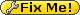#Xevelabs Product Documentation

Home of the USB2AX

### Site Tools

product:usb2lds:parameters

This is an old revision of the document!

## USB2LDS: Setting parameters

The USB2LDS has a set of parameters that can be changed to adapt the behaviour of the LIDAR control. This page only relates to the parameters of the USB2LDS, see Talking directly to the LDS if you want to get send commands to the LDS itself.

### Putting the USB2LDS in setup mode

To set the parameters, the USB2LDS has to be in setup mode.

### Commands

All the setup can be managed with single-character commands.

For most numerical values, there is a common “delta” parameter that is used to make it easy to adjust very different ranges. It's like selecting the digit you want to go up or down in the parameter you are adjusting, and it can go all the way up (>10000) or down(<0.0001).

Command Description
a Display complete setup
p Decrease PID coefficient P - delta (P-=delta)
P Increase PID coefficient P + delta (P+=delta)
i Decrease PID coefficient I - delta (I-=delta)
I Increase PID coefficient I + delta (I+=delta)
d Decrease PID coefficient D - delta (D-=delta)
D Increase PID coefficient D + delta (D+=delta)
+ Set delta to the upper decade (delta*=10)
- Set delta to the lower decade (delta/=10)
r Decrease turn rate setpoint by 64 (RPM-=64)
R Increase turn rate setpoint by 64 (RPM+=64)
0 Set Feedforward mode to NONE
1 Set Feedforward mode to FIXED
2 Set Feedforward mode to PROPORTIONAL
f Decrease Feedforward by 1 (feedforward-=1)
F Increase Feedforward by 1 (feedforward+=1)
c Decrease Feedforward coefficient by delta (feedforward_coeff-=delta)
C Increase Feedforward coefficient by delta (feedforward_coeff+=delta)
s Save parameters to EEPROM (careful, there is no confirmation!)
m Stop the motor
M Start the motor
g Set Display mode to NONE
G Set Display mode to GRAPH (Not Implemented Yet)
v Set Display mode to VALUES
' ' (space) Set PWM to 200 to make the motor spin whatever the computation. Useful to reset the turn rate to a known value when parameters made it go too fast or too slow for the PID to stabilize.

#### Memory

There are 3 types of memory on the USB2LDS, and settings can be managed

RAM : short term stoarge that is used for current operations.
EEPROM : long term storage that is not wiped out when power is removed. It can be modified by user actions.
FLASH : Long term storage that is not wiped out when power is removed. It can be changed only by re-programming the board, so there is no risk of losing it.

• At startup ⇒ EEPROM to RAM
• all the modifications done with commands happen in RAM
• Save ⇒ RAM to EEPROM
• Load default ⇒ FLASH to RAM

#### PID coefficients

• P, I and D cannot be negative and are limited to 0.

Default are#### turn rate setpoint

The turn rate of the LDS can be selected by adjusting the turn rate setpoint. Its unit is in RPM *64, meaning that a setpoint value of 19200 will be interpreted as 300 RPM (300*64 = 19200) or 5 rotation per second (5*60*64=19200).

#### Feedforward

The system accepts a feedforward value, that is to say a value that will be added automatically to the output in an effort to lighten the load of the PID. Its presence is there to counteract known and constant disturbance like the friction of the rotating assembly.

• NONE: no feedforward
• FIXED : feedforward is a fixed value (adjused with 'f' and 'F')
• PROPORTIONAL : feedforward is a percentage of the RPM setpoint (adjusted with 'c'and 'C')

Default is